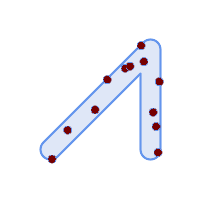## Name

ST_GeneratePoints — Generates a multipoint of random points contained in a Polygon or MultiPolygon.

## Synopsis

`geometry ST_GeneratePoints(`geometry g, integer npoints, integer seed = 0`)`;

## Descrição

ST_GeneratePoints generates a multipoint consisting of a given number of pseudo-random points which lie within the input area. The optional `seed` is used to regenerate a deterministic sequence of points, and must be greater than zero.

## ExemplosGenerated a multipoint consisting of 12 Points overlaid on top of original polygon using a random seed value 1996

```SELECT ST_GeneratePoints(geom, 12, 1996)
FROM (
SELECT ST_Buffer(
ST_GeomFromText(
'LINESTRING(50 50,150 150,150 50)'),
10, 'endcap=round join=round') AS geom
) AS s;```

Given a table of polygons s, return 12 individual points per polygon. Results will be different each time you run.

```SELECT s.id, dp.path AS pt_id, dp.geom
FROM s, ST_DumpPoints(ST_GeneratePoints(s.geom,12)) AS dp;```